# Bouncing ball facts for kids

Kids Encyclopedia Facts

The physics of a bouncing ball concerns the physical behaviour of bouncing balls, particularly its motion before, during, and after impact against the surface of another body. Several aspects of a bouncing ball's behaviour serve as an introduction to mechanics in high school or undergraduate level physics courses. However, the exact modelling of the behaviour is complex and of interest in sports engineering.

The motion of a ball is generally described by projectile motion (which can be affected by gravity, drag, the Magnus effect, and buoyancy), while its impact is usually characterized through the coefficient of restitution (which can be affected by the nature of the ball, the nature of the impacting surface, the impact velocity, rotation, and local conditions such as temperature and pressure). To ensure fair play, many sports governing bodies set limits on the bounciness of their ball and forbid tampering with the ball's aerodynamic properties. The bounciness of balls has been a feature of sports as ancient as the Mesoamerican ballgame.

## Forces during flight and effect on motion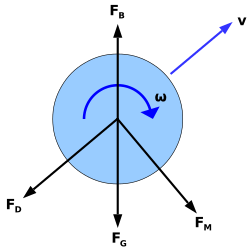The forces acting on a spinning ball during its flight are the gravitational force (FG), the drag force (FD), the Magnus force (FM), and the buoyant force (FB).

The motion of a bouncing ball obeys projectile motion. Many forces act on a real ball, namely the gravitational force (FG), the drag force due to air resistance (FD), the Magnus force due to the ball's spin (FM), and the buoyant force (FB). In general, one has to use Newton's second law taking all forces into account to analyze the ball's motion: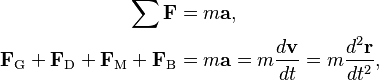\begin{align} \sum \mathbf{F} & = m\mathbf{a}, \\ \mathbf{F}_\text{G} + \mathbf{F}_\text{D} + \mathbf{F}_\text{M} + \mathbf{F}_\text{B} & = m \mathbf{a} = m \frac{d\mathbf{v}}{dt} = m\frac{d^2\mathbf{r}}{dt^2}, \end{align}

where m is the ball's mass. Here, a, v, r represent the ball's acceleration, velocity, and position over time t.

### Gravity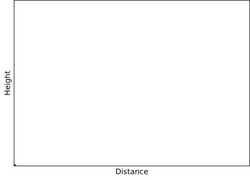Trajectory of a ball bouncing at an angle of 70° after impact without , with Stokes drag     , and with .

The gravitational force is directed downwards and is equal to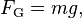$F_\text{G} = mg,$

where m is the mass of the ball, and g is the gravitational acceleration, which on Earth varies between 9.764 m/s2 and 9.834 m/s2. Because the other forces are usually small, the motion is often idealized as being only under the influence of gravity. If only the force of gravity acts on the ball, the mechanical energy will be conserved during its flight. In this idealized case, the equations of motion are given by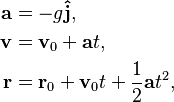\begin{align} \mathbf{a} & = -g \mathbf{\hat{j}}, \\ \mathbf{v} & = \mathbf{v}_\text{0} + \mathbf{a}t, \\ \mathbf{r} & = \mathbf{r}_0 + \mathbf{v}_0 t + \frac{1}{2}\mathbf{a}t^2, \end{align}

where a, v, and r denote the acceleration, velocity, and position of the ball, and v0 and r0 are the initial velocity and position of the ball, respectively.

More specifically, if the ball is bounced at an angle θ with the ground, the motion in the x- and y-axes (representing horizontal and vertical motion, respectively) is described by

 x-axis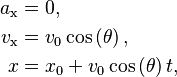\begin{align} a_\text{x} & = 0, \\ v_\text{x} & = v_0 \cos \left(\theta \right), \\ x & = x_0 + v_0 \cos \left( \theta \right) t, \end{align} y-axis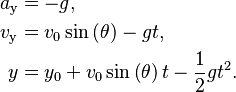\begin{align} a_\text{y} & = -g, \\ v_\text{y} & = v_0 \sin \left(\theta \right) -gt, \\ y & = y_0 + v_0 \sin \left( \theta \right) t -\frac{1}{2}gt^2. \end{align}

The equations imply that the maximum height (H) and range (R) and time of flight (T) of a ball bouncing on a flat surface are given by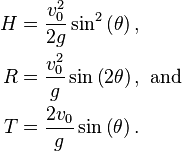\begin{align} H & = \frac{v_0^2}{2g}\sin^2\left(\theta\right), \\ R &= \frac{v_0^2}{g}\sin\left(2\theta\right),~\text{and} \\ T &= \frac{2v_0}{g} \sin \left(\theta \right). \end{align}

Further refinements to the motion of the ball can be made by taking into account air resistance (and related effects such as drag and wind), the Magnus effect, and buoyancy. Because lighter balls accelerate more readily, their motion tends to be affected more by such forces.

### Drag

Air flow around the ball can be either laminar or turbulent depending on the Reynolds number (Re), defined as: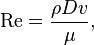$\text{Re} = \frac{\rho D v}{\mu},$

where ρ is the density of air, μ the dynamic viscosity of air, D the diameter of the ball, and v the velocity of the ball through air. At a temperature of 20 °C, ρ = 1.2 kg/m3 and μ = 1.8×10−5 Pa·s.

If the Reynolds number is very low (Re < 1), the drag force on the ball is described by Stokes' law: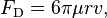$F_\text{D} = 6 \pi \mu r v,$

where r is the radius of the ball. This force acts in opposition to the ball's direction (in the direction of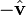$\textstyle -\hat \mathbf{v}$). For most sports balls, however, the Reynolds number will be between 104 and 105 and Stokes' law does not apply. At these higher values of the Reynolds number, the drag force on the ball is instead described by the drag equation: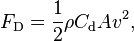$F_\text{D} = \frac{1}{2} \rho C_\text{d} A v^2,$

where Cd is the drag coefficient, and A the cross-sectional area of the ball.

Drag will cause the ball to lose mechanical energy during its flight, and will reduce its range and height, while crosswinds will deflect it from its original path. Both effects have to be taken into account by players in sports such as golf.

### Magnus effect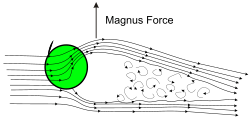The Magnus force acting on a ball with backspin. The curly flow lines represent a turbulent wake. The airflow has been deflected in the direction of spin.
In table tennis, a skilled player can exploit the ball's spin to affect the trajectory of the ball during its flight and its reaction upon impact with a surface. With topspin, the ball reaches maximum height further into its flight (1) and then curves abruptly downwards (2). The impact propels the ball forward (3) and will tend to bounce upwards when impacting the opposing player's paddle. The situation is opposite in the case of backspin.

The spin of the ball will affect its trajectory through the Magnus effect. According to the Kutta–Joukowski theorem, for a spinning sphere with an inviscid flow of air, the Magnus force is equal to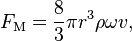$F_\text{M} = \frac{8}{3} \pi r^3 \rho \omega v,$

where r is the radius of the ball, ω the angular velocity (or spin rate) of the ball, ρ the density of air, and v the velocity of the ball relative to air. This force is directed perpendicular to the motion and perpendicular to the axis of rotation (in the direction of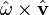$\textstyle \hat \mathbf{\omega} \times \hat \mathbf{v}$). The force is directed upwards for backspin and downwards for topspin. In reality, flow is never inviscid, and the Magnus lift is better described by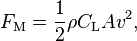$F_\text{M}=\frac{1}{2}\rho C_\text{L} A v^2,$

where ρ is the density of air, CL the lift coefficient, A the cross-sectional area of the ball, and v the velocity of the ball relative to air. The lift coefficient is a complex factor which depends amongst other things on the ratio /v, the Reynolds number, and surface roughness. In certain conditions, the lift coefficient can even be negative, changing the direction of the Magnus force (reverse Magnus effect).

In sports like tennis or volleyball, the player can use the Magnus effect to control the ball's trajectory (e.g. via topspin or backspin) during flight. In golf, the effect is responsible for slicing and hooking which are usually a detriment to the golfer, but also helps with increasing the range of a drive and other shots. In baseball, pitchers use the effect to create curveballs and other special pitches.

Ball tampering is often illegal, and is often at the centre of cricket controversies such as the one between England and Pakistan in August 2006. In baseball, the term 'spitball' refers to the illegal coating of the ball with spit or other substances to alter the aerodynamics of the ball.

### Buoyancy

Any object immersed in a fluid such as water or air will experience an upwards buoyancy. According to Archimedes' principle, this buoyant force is equal to the weight of the fluid displaced by the object. In the case of a sphere, this force is equal to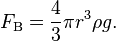$F_\text{B} = \frac{4}{3}\pi r^3 \rho g.$

The buoyant force is usually small compared to the drag and Magnus forces and can often be neglected. However, in the case of a basketball, the buoyant force can amount to about 1.5% of the ball's weight. Since buoyancy is directed upwards, it will act to increase the range and height of the ball.

## Impact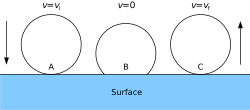The compression (A→B) and decompression (B→C) of a ball impacting against a surface. The force of impact is usually proportional to the compression distance, at least for small compressions, and can be modelled as a spring force.

When a ball impacts a surface, the surface recoils and vibrates, as does the ball, creating both sound and heat, and the ball loses kinetic energy. Additionally, the impact can impart some rotation to the ball, transferring some of its translational kinetic energy into rotational kinetic energy. This energy loss is usually characterized (indirectly) through the coefficient of restitution (or COR, denoted e):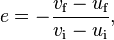$e = -\frac{v_\text{f} - u_\text{f}}{v_\text{i} - u_\text{i}},$

where vf and vi are the final and initial velocities of the ball, and uf and ui are the final and initial velocities impacting surface, respectively. In the specific case where a ball impacts on an immovable surface, the COR simplifies to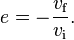$e = -\frac{v_\text{f}}{v_\text{i}}.$

For a ball dropped against a floor, the COR will therefore vary between 0 (no bounce, total loss of energy) and 1 (perfectly bouncy, no energy loss). A COR value below 0 or above 1 is theoretically possible, but would indicate that the ball went through the surface (e < 0), or that the surface was not "relaxed" when the ball impacted it (e > 1), like in the case of a ball landing on spring-loaded platform.

To analyze the vertical and horizontal components of the motion, the COR is sometimes split up into a normal COR (ey), and tangential COR (ex), defined as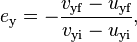$e_\text{y} = -\frac{v_\text{yf} - u_\text{yf}}{v_\text{yi} - u_\text{yi}},$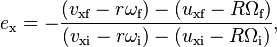$e_\text{x} = -\frac{(v_\text{xf}-r\omega_\text{f})-(u_\text{xf}-R\Omega_\text{f})}{(v_\text{xi}-r\omega_\text{i})-(u_\text{xi}-R\Omega_\text{i})},$

where r and ω denote the radius and angular velocity of the ball, while R and Ω denote the radius and angular velocity the impacting surface (such as a baseball bat). In particular is the tangential velocity of the ball's surface, while is the tangential velocity of the impacting surface. These are especially of interest when the ball impacts the surface at an oblique angle, or when rotation is involved.

For a straight drop on the ground with no rotation, with only the force of gravity acting on the ball, the COR can be related to several other quantities by: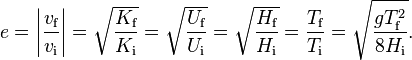$e = \left|\frac{v_\text{f}}{v_\text{i}}\right| = \sqrt{\frac{K_\text{f}}{K_\text{i}}} = \sqrt{\frac{U_\text{f}}{U_\text{i}}} = \sqrt{\frac{H_\text{f}}{H_\text{i}}} = \frac{T_\text{f}}{T_\text{i}} =\sqrt{\frac{gT^2_\text{f}}{8H_\text{i}}}.$

Here, K and U denote the kinetic and potential energy of the ball, H is the maximum height of the ball, and T is the time of flight of the ball. The 'i' and 'f' subscript refer to the initial (before impact) and final (after impact) states of the ball. Likewise, the energy loss at impact can be related to the COR by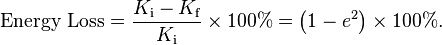$\text{Energy Loss} = \frac{{K_\text{i}}-{K_\text{f}}}{K_\text{i}} \times 100\% = \left( 1 - e^2 \right) \times 100\%.$

The COR of a ball can be affected by several things, mainly

• the nature of the impacting surface (e.g. grass, concrete, wire mesh)
• the material of the ball (e.g. leather, rubber, plastic)
• the pressure inside the ball (if hollow)
• the amount of rotation induced in the ball at impact
• the impact velocity

External conditions such as temperature can change the properties of the impacting surface or of the ball, making them either more flexible or more rigid. This will, in turn, affect the COR. In general, the ball will deform more at higher impact velocities and will accordingly lose more of its energy, decreasing its COR.

### Spin and angle of impact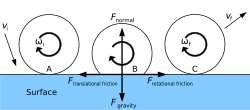The forces acting on a spinning ball during impact are the force of gravity, the normal force, and the force of friction (which has in general both a 'translational' and a 'rotational' component). If the surface is angled, the force of gravity would be at an angle from the surface, while the other forces would remain perpendicular or parallel to the surface.

Upon impacting the ground, some translational kinetic energy can be converted to rotational kinetic energy and vice versa depending on the ball's impact angle and angular velocity. If the ball moves horizontally at impact, friction will have a 'translational' component in the direction opposite to the ball's motion. In the figure, the ball is moving to the right, and thus it will have a translational component of friction pushing the ball to the left. Additionally, if the ball is spinning at impact, friction will have a 'rotational' component in the direction opposite to the ball's rotation. On the figure, the ball is spinning clockwise, and the point impacting the ground is moving to the left with respect to the ball's center of mass. The rotational component of friction is therefore pushing the ball to the right. Unlike the normal force and the force of gravity, these frictional forces will exert a torque on the ball, and change its angular velocity (ω).

Three situations can arise:

1. If a ball is propelled forward with backspin, the translational and rotational friction will act in the same directions. The ball's angular velocity will be reduced after impact, as will its horizontal velocity, and the ball is propelled upwards, possibly even exceeding its original height. It is also possible for the ball to start spinning in the opposite direction, and even bounce backwards.
2. If a ball is propelled forward with topspin, the translational and rotational friction act will act in opposite directions. What exactly happens depends on which of the two components dominate.
1. If the ball is spinning much more rapidly than it was moving, rotational friction will dominate. The ball's angular velocity will be reduced after impact, but its horizontal velocity will be increased. The ball will be propelled forward but will not exceed its original height, and will keep spinning in the same direction.
2. If the ball is moving much more rapidly than it was spinning, translational friction will dominate. The ball's angular velocity will be increased after impact, but its horizontal velocity will be decreased. The ball will not exceed its original height and will keep spinning in the same direction.

If the surface is inclined by some amount θ, the entire diagram would be rotated by θ, but the force of gravity would remain pointing downwards (forming an angle θ with the surface). Gravity would then have a component parallel to the surface, which would contribute to friction, and thus contribute to rotation.

In racquet sports such as table tennis or racquetball, skilled players will use spin (including sidespin) to suddenly alter the ball's direction when it impacts surface, such as the ground or their opponent's racquet. Similarly, in cricket, there are various methods of spin bowling that can make the ball deviate significantly off the pitch.

### Non-spherical balls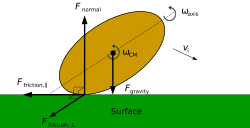The forces acting on a gridiron football ball or rugby ball at impact are the force of gravity, the normal force, and the force of friction. Friction will normally have a 'longitudinal' component due to the ball's velocity and 'tumbling' spin and a 'sideways' component due to the 'on-axis' spin of the ball induced by the throw.

The bounce of an oval-shaped ball (such as those used in gridiron football or rugby football) is in general much less predictable than the bounce of a spherical ball. Depending on the ball's alignment at impact, the normal force can act ahead or behind the centre of mass of the ball, and friction from the ground will depend on the alignment of the ball, as well as its rotation, spin, and impact velocity. Where the forces act with respect to the centre of mass of the ball changes as the ball rolls on the ground, and all forces can exert a torque on the ball, including the normal force and the force of gravity. This can cause the ball to bounce forward, bounce back, or sideways. Because it is possible to transfer some rotational kinetic energy into translational kinetic energy, it is even possible for the COR to be greater than 1, or for the forward velocity of the ball to increase upon impact.

### Multiple stacked balls

A popular demonstration involves the bounce of multiple stacked balls. If a tennis ball is stacked on top of a basketball, and the two of them are dropped at the same time, the tennis ball will bounce much higher than it would have if dropped on its own, even exceeding its original release height. The result is surprising as it apparently violates conservation of energy. However, upon closer inspection, the basketball does not bounce as high as it would have if the tennis ball had not been on top of it, and transferred some of its energy into the tennis ball, propelling it to a greater height.

The usual explanation involves considering two separate impacts: the basketball impacting with the floor, and then the basketball impacting with the tennis ball. Assuming perfectly elastic collisions, the basketball impacting the floor at 1 m/s would rebound at 1 m/s. The tennis ball going at 1 m/s would then have a relative impact velocity of 2 m/s, which means it would rebound at 2 m/s relative to the basketball, or 3 m/s relative to the floor, and triple its rebound velocity compared to impacting the floor on its own. This implies that the ball would bounce to 9 times its original height. In reality, due to inelastic collisions, the tennis ball will increase its velocity and rebound height by a smaller factor, but still will bounce faster and higher than it would have on its own.

While the assumptions of separate impacts is not actually valid (the balls remain in close contact with each other during most of the impact), this model will nonetheless reproduce experimental results with good agreement, and is often used to understand more complex phenomena such as the core collapse of supernovae, or gravitational slingshot manoeuvres.

## Sport regulations

See also: Regulation of sport

Several sports governing bodies regulate the bounciness of a ball through various ways, some direct, some indirect.

• AFL: Regulates the gauge pressure of the football to be between 62 kPa and 76 kPa.
• FIBA: Regulates the gauge pressure so the basketball bounces between 1200 mm and 1400 mm (top of the ball) when it is dropped from a height of 1800 mm (bottom of the ball). This roughly corresponds to a COR of 0.727 to 0.806.
• FIFA: Regulates the gauge pressure of the soccer ball to be between of 0.6 atm and 1.1 atm at sea level (61 to 111 kPa).
• FIVB: Regulates the gauge pressure of the volleyball to be between 0.30 kgF/cm2 to 0.325 kgF/cm2 (29.4 to 31.9 kPa) for indoor volleyball, and 0.175 kgF/cm2 to 0.225 kgF/cm2 (17.2 to 22.1 kPa) for beach volleyball.
• ITF: Regulates the height of the tennis ball bounce when dropped on a "smooth, rigid and horizontal block of high mass". Different types of ball are allowed for different types of surfaces. When dropped from a height of 100 inches (254 cm), the bounce must be 54–60 in (137–152 cm) for Type 1 balls, 53–58 in (135–147 cm) for Type 2 and Type 3 balls, and 48–53 in (122–135 cm) for High Altitude balls. This roughly corresponds to a COR of 0.735–0.775 (Type 1 ball), 0.728–0.762 (Type 2 & 3 balls), and 0.693–0.728 (High Altitude balls) when dropped on the testing surface.
• ITTF: Regulates the playing surface so that the table tennis ball bounces approximately 23 cm when dropped from a height of 30 cm. This roughly corresponds to a COR of about 0.876 against the playing surface.
• NBA: Regulates the gauge pressure of the basketball to be between 7.5 and 8.5 psi (51.7 to 58.6 kPa).
• NFL: Regulates the gauge pressure of the American football to be between 12.5 and 13.5 psi (86 to 93 kPa).
• R&A/USGA: Limits the COR of the golf ball directly, which should not exceed 0.83 against a golf club.

The pressure of an American football was at the center of the deflategate controversy. Some sports do not regulate the bouncing properties of balls directly, but instead specify a construction method. In baseball, the introduction of a cork-based ball helped to end the dead-ball era and trigger the live-ball era.Bouncing ball Facts for Kids. Kiddle Encyclopedia.